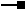# IXMLSerializeData2 Interface

## Members

Name DescriptionAddBinary Adds element value as an array of bytes.AddBoolean Adds element value as a boolean.AddByte Adds element value as a byte.AddDate Adds element value as a date.AddDouble Adds element value as a double.AddFloat Adds element value as a float.AddInt64 Adds element value as an int64.AddInteger Adds element value as an integer.AddObject Adds element value as an object.AddShort Adds element value as a short.AddString Adds element value as a string.AddVariant Adds element value as a variant.Count Number of XML elements.Find Finds an XML element by name.GetBinary Obtains element value as an array of bytes.GetBoolean Obtains element value as a boolean.GetByte Obtains element value as a byte.GetDate Obtains element value as a date.GetDouble Obtains element value as a double.GetFlag Obtains the value for a serialization flag.GetFloat Obtains element value as a float.GetInt64 Obtains element value as an int64.GetInteger Obtains element value as an integer.GetObject Obtains element value as an object instance.GetShort Obtains element value as a short.GetString Obtains element value as a string.GetVariant Obtains element value as a variant.Properties Properties for serialization and deserialization.SetFlag Writes the value for a serialization flag.TypeName XML type of the object.TypeName XML type of the object.TypeNamespaceURI XML type namespace of the object.TypeNamespaceURI XML type namespace of the object.

Adds element value as an int64.

``````Public Sub AddInt64 ( _
ByVal Name As String, _
ByVal Value As Long _
)
``````
``````public void AddInt64 (
string Name,
long Value
);
``````

### IXMLSerializeData2.GetInt64 Method

Obtains element value as an int64.

``````Public Function GetInt64 ( _
ByVal Index As Integer _
) As Long
``````
``````public long GetInt64 (
int Index
);
``````

## Inherited Interfaces

Interfaces Description
IXMLSerializeData Provides access to members that serialize and deserialize data from XML.

## Classes that implement IXMLSerializeData2

Classes Description